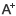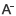# 浙教版小学六年级下数学配套训练册答案日期：2020-05-08     来源：www.zhixueshuo.com    作者：智学网    浏览：514    评论：0

1、直线 无

2、

=πdS =πr

3、

1、（2 + 2.8）× 2 ÷ 2 = 4.8（平方厘米）
1 × 3.14 = 3.14（平方厘米）
4.8 – 3.14 = 1.66（平方厘米）
2、8 × 8 = 64（平方厘米） 面积：64 - 4 × 3.14 = 13.76（平方厘米） 周长：8 × 3.14 = 25.12（厘米）

1、× 2、× 3、√ 4、× 5、× 6、√

1、B 2、B 3、C 4、C 5、C 6、B

8 10

4

1、r = 18.84 ÷ 3.14 ÷ 2 = 3（厘米）S = 3 × 3.14 = 28.26（平方厘米）
2、3 × 8 = 24（厘米）
3、（45 + 5）÷ 2 = 25（厘米）25 × 3.14 = 1962.5（平方厘米）
4、题目略
（1）5 × 5 = 25（平米）
（2）5 × 5 × 2 = 50（立方米）
（3）5 × 5 +（5 × 2 + 5 × 2）× 2 = 65（平米）
5、12 ÷ 4 = 3（厘米） 高12厘米，3 × 3 × 12 = 108（立方厘米）

0相关评论